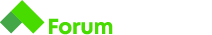# Calculate Change C#

Здравейте!

Някой може ли да даде насоки за тази задача.

# Calculate Change

Write a program that determines the least amount of coins needed for a cashier to return change.

For example, if the price of something is 0.76 leva and the customer has paid 1 leva, the least amount of coins is `1 x 20 stotinki` and `2 x 2 stotinki` .

Use the coins of 1 lev, 50, 20, 10, 5, 2 and 1 stotinki.

## Input

• On the first line, you will receive the price in leva.
• On the second line, you will receive how much the customer has paid in leva.

## Output

• There is a variable amount of output lines.
• Print each required denomination on a new line, ordered from highest to lowest.

## Input

``````0.76
1
``````

## Output

``````1 x 20 stotinki
2 x 2 stotinki
``````

## Input

``````1.92
5
``````

## Output

``````3 x 1 lev
1 x 5 stotinki
1 x 2 stotinki
1 x 1 stotinka
``````

Превърни и двете подадени стоийности в стотинки. Вадиш от това което е дадено сметката (100-76 = 24 стотинки ресто) и след това проверяваш дали рестото е над 100 (int leva = change / 100) след което правиш модулно деление c%100 за да разкараш 1-вото число и продължаваш със останалите.

``````double price = double.Parse(Console.ReadLine());

double change = paid - price;

change *= 100;

if(change >= 100)
{
Console.WriteLine((int)change / 100 + " x 1 lev");
change %= 100;
}
``````

Ето началото , пробвай сама нататък, ако пак не става , пиши*= ; %= ; ((int)change ;
Какво означават тези операнти ? Каква е целта на int в () ? Аз съм от по- начинаещитеИзтрих си поста понеже бях сгафил някъде, нещо не работеше както тряябва

``````int a = 5 ;
a *= 10  е все едно a = a * 10 , просто е съкратен формат
(int) се нарича кастване , понеже в началото инпута е double , за да може да се въведе 1.92 , но след това ако искам да принтирам само цялото число го "каст"-вам към инт , понеже ако имам
3.14 и му кажа (int) ще принтира 3
``````

Малко съм разсеян, тествал съм си кода на друга задача и ми даваше грешен отговор. Правилно е всичко , ако пак има нещо , питай смело

Защо делим int change на 100 и защо е това допълнение change change %= 100?

Да вземем за пример 1.92 сметка и 5 лева платени
Както предложих да ги превърнем в стотинки , че се смята по-лесно
1.92 * 100 = 192 стотинки
5 * 100 = 500 стотинки
500 - 192 = 308 стотинки - 3 лева и 8 стотинки - ресто
1-во проверяваме дали има поне 1 лев ресто , затова проверяваме дали change >= 100 и ако е така делиим на 100 но без да променяме стойността, а само я принтираме.
308 / 100 = 3.08 , ако го няма това (int) ще се принтира 3.08 , понеже променливата е от тип double. Би трябвало да знаеш , че int се ползва само за цели числа и по този начин форсирам кода да принтира цяло число и дори да имам 3.99 пак ще принтира 3.
Рестото все още е 308 стотинки , промени не са правени.
change %= 100 е еквивалент на change = change % 100
Тук вече променяме числото.
308 % 100 = 8 стотинки. // това е делене с остатък.
Ако беше примерно 355 , щеше да бъде 355 % 100 = 55 и т.н.
След като обработим “лев”-овете , продължаваме към стотинките на същият принцип.

Надявам се това да помогна.

1 Like

Решението супер си работи! Искрени благодарностиПак заповядай. Даде ли ти 100 точки ?

Да. Сега като го гледам не е нищо сложно , просто една идея нови знания

1 Like